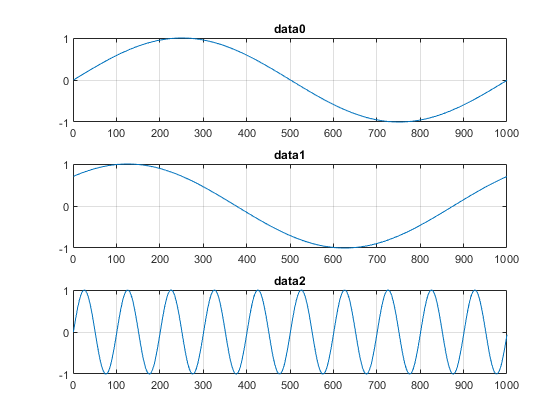Documentation

Generate Continuous and Background Signals Using NI Devices

This example shows how to generate analog output data using non-blocking commands. This allows you to continue working in the MATLAB command window during the generation. This is called background generation. Use foreground generation to cause MATLAB to wait for the entire data generation to complete before you can execute your next command. Learn more about foreground generation in Generate Signals on NI devices that Output Voltage.

Create and Configure the Session Object

Use daq.getDevices to display a list of devices available to your machine and MATLAB®. For this example use the NI-9263 with device ID 'cDAQ1Mod2'. Output data on 3 channels at a rate of 10000 scans per second.

s = daq.createSession('ni');

s.Rate = 10000;

Create Synchronized Signals

Generate output signals by creating a pattern of data that is repeatedly queued to the output device. The data for each channel is column based and the output signals are synchronized to each other.

Create 3 waveforms:

• data0: 1 cycle of a sine wave

• data1: 1 cycle of a sine wave with a 45 degree phase lag

• data2: 10 cycles of a sine wave

data0 = sin(linspace(0, 2*pi, 1001))';
data1 = sin(linspace(0, 2*pi, 1001) + pi/4)';
data2 = sin(linspace(0, 2*pi*10, 1001))';

The above code creates a 1001 point waveform. This is because linspace includes the endpoints, we will have a data point for sin(0) and a data point for sin(2*pi). To maintain a coherent signal we delete the last point to end up with 1000 points per waveform.

data0(end) = [];
data1(end) = [];
data2(end) = [];

Note that we setup the session to run at a rate of 10,000 scans per second. Since each cycle of the output sine waves is 1000 points, we expect to observe data0 and data1 as 10Hz sine waves, and data2 as a 100Hz sine wave.

subplot(3,1,1)
plot(data0)
title('data0')
grid on
subplot(3,1,2)
plot(data1)
title('data1')
grid on;
subplot(3,1,3)
plot(data2)
title('data2')
grid on;Queue the Output Data and Start Background Generation

Before starting a continuous generation, queue half a second of data to the analog output device's buffer. Then call startBackground. The session expects the queued output data to be column based, with a column of data for each output channel added to your session.

The startBackground command starts the generation and returns control to the command line immediately, allowing you to do other operations in MATLAB while the generation is running in the background.

queueOutputData(s,repmat([data0, data1, data2], 5, 1));
s.startBackground();

You can run other MATLAB commands while the generation is running, or optionally call wait with no argument, which defaults to an infinite wait time. The wait command returns as soon as the background generation completes.

s.wait()

Create a Function to Continuously Queue Output Data

When you generate data in the background, you can provide the acquisition session directions to handle the outgoing data, using listeners and events. For example, you can continuously generate the output signal by queuing output data repeatedly. A DataRequired event occurs indicating that we need to queue more data to the device. A listener can respond to that event and initiate specified function.

Use addListener to add an anonymous function to the session and assign it to the DataRequired event. The event calls the anonymous function repeatedly to queue more data to the output queue, thus creating continuous signal generation.

Save the listener handle in the variable lh that you will delete later.

lh = addlistener(s,'DataRequired', ...
@(src,event) src.queueOutputData([data0, data1, data2]));

Setup the Session and Start the Continuous Generation

For continuous generation:

• Set the session to continuous mode

• Queue the output data

• call startBackground

s.IsContinuous = true;
queueOutputData(s,repmat([data0, data1, data2], 5, 1));
s.startBackground();

Note: In continuous generation, always feed adequate data to the device. If you output data at a rate much higher than the amount of data you are queuing to the device, the device may run out of data to output, resulting in an underflow error. Refer to the CompactDAQ documentation for more details.

Stop the Continuous Background Generation

Continuous generation runs in parallel with other operations in MATLAB. Explicitly call stop to end the background generation. Delete the listener in the end.

s.stop();
delete(lh);

Generate Output Data Dynamically Using MATLAB Functions

To dynamically generate the output data using a MATLAB function instead of a simple one line anonymous function add a listener event handler.

lh = addlistener(s,'DataRequired', @myFunction);
function myFunction(src, event)
% Your algorithm to dynamically generate the data
%
queueOutputData(src,[data0, data1, data2]);
end
Data Acquisition Toolbox DocumentationGet trial now